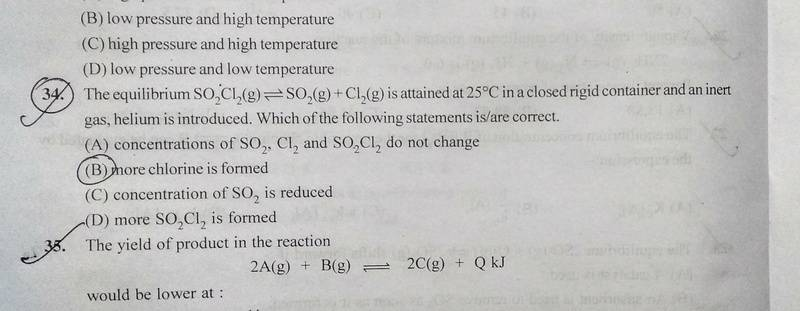# Why doesn't the amount of Cl increase?

Gold Member

## Homework Statement## Homework Equations

Formula of Kp and Kc

## The Attempt at a Solution

You will easily be able to identify that the reaction will proceed in the forward direction when some inert gas is introduced.
So,my question is that why won't the amount of chlorine formed increase in that process(because the reaction is proceeding forward)?

I will be thankful for any help!

#### Attachments

Gold Member
To my knowledge, if you insert some inert gas the partial pressure of the components increases and so the inverse reaction in favored. Intuitively think about it: you have two separate molecules, if you try to push them together then it will be reasonable that they will react.

Gold Member
You will easily be able to identify that the reaction will proceed in the forward direction when some inert gas is introduced.
The gases are at equilibrium.
Sorry, but I cannot identify that the reaction will proceed in the forward direction when an inert gas is introduced.
Perhaps you could explain your reasoning, through the use of the formula of Kp

Gold Member
I think the following. I don't know if it is rigorous enough for you.

Consider the gas-phase reaction ##A → B + C## $$K = \frac {P x_B P x_C} {P x_A} = P \frac {x_B x_C} {x_A}$$ if ##P## increases then ##\frac {x_B x_C} {x_A}## must decrease to keep ##K## constant (remember that ##K## is a function of the Temperature only). Introducing an inert gas obviously increase the pressure if you keep the volume constant.

So more ##A## is produced

Gold Member
I think the following. I don't know if it is rigorous enough for you.

Consider the gas-phase reaction ##A → B + C## $$K = \frac {P x_B P x_C} {P x_A} = P \frac {x_B x_C} {x_A}$$ if ##P## increases then ##\frac {x_B x_C} {x_A}## must decrease to keep ##K## constant (remember that ##K## is a function of the Temperature only). Introducing an inert gas obviously increase the pressure if you keep the volume constant.

So more ##A## is produced
But if you introduce an inert gas, the mole fractions of the individual gasses will also decrease.

Gold Member
When an inert gas is added to the system in equilibrium at constant volume V, the total pressure will increase, but the partial pressures pi of the reacting components remain constant:

pi = ni RT / V with ni the moles of gas component i

Hence, there will be no effect on the equilibrium.

•Chestermiller, dRic2 and epenguin
Gold Member
My mistake, I'm sorry.